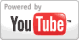Area of a Triangle - Geometry Lesson
Grade: 6 - 12 | In this lesson, learn the formula for the area of a triangle, and solve problems using this formula. Note that the lesson uses right triangle formulas and trigonometry extensively.Add to My DashboardGeometry### Search Videos & Games